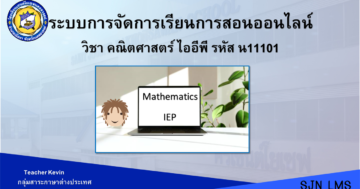# Mathematics IEP P.1 2nd Semester## เกี่ยวกับคอร์ส

Students are able to tell the numbers of things and represent things in determined

numbers, read and write Hindu-Arabic and Thai numbers not exceeding 100 and 0; compare the

numbers not exceeding 100 and O by using the sign =; arrange 3 – 5 numbers not exceeding

100 and 0; find the value of the variable in number sentence of adding and subtracting of numbers

not exceeding 100 and O; show the working of the problem in adding and subtracting of the

numbers not exceeding 100 and 0; tell the missing numbers in patterns of numbers which

increase or decrease by 1s and 10s; tell the missing geometries in the repeated pattern of

geometries and pictures which there are 2 repeated in the patterns; measure and compare the

length in centimeter and meter, the weight in kilogram and kheed; differentiate the pictures of

triangles, quadrilaterals, circles, ovals, cubes, spheres, cylinders, and cones; and use the

information from the pictogram to find the answer for the problem when giving 1 picture per

unit.

These are for the students to learn, develop the skills on calculation, problem solving,

reasoning, and mathematical interpretation to creatively use in daily life; appreciate and have

good attitude towards mathematics. Students can systematically and thoroughly work with

responsibility, judgment, and self-confidence.

00:00
00:00

00:00

00:00

00:00
00:00

00:00
00:00
00:00
00:00

### Student Ratings & ReviewsNo Review Yet